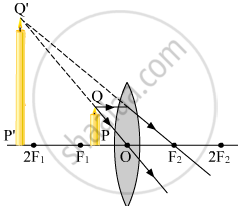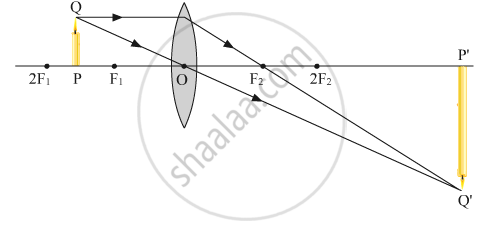Share

# "A lens can form a magnified erect image as well as magnified inverted image of an object placed in front of it." State the nature of this lens and draw ray diagrams to justify the above statement. Mark the positions of O, F and 2F in the diagram. - CBSE Class 10 - Science

ConceptRefraction of Light Image Formation by Lenses - Convex Lens

#### Question

"A lens can form a magnified erect image as well as magnified inverted image of an object placed in front of it." State the nature of this lens and draw ray diagrams to justify the above statement. Mark the positions of O, F and 2F in the diagram.

#### Solution

The lens is a convex in nature. Thus, it is a convex lens.

When the object is placed between the focus F1 and optical centre O a magnified erect image is formed as shown below.When the object is placed between the focus F1 and 2F1 a magnified inverted image​ is formed as shown below.​Is there an error in this question or solution?

#### Video TutorialsVIEW ALL 

Solution "A lens can form a magnified erect image as well as magnified inverted image of an object placed in front of it." State the nature of this lens and draw ray diagrams to justify the above statement. Mark the positions of O, F and 2F in the diagram. Concept: Refraction of Light - Image Formation by Lenses - Convex Lens.
S